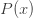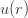# Sampling from a Probability Distribution

Posted on the 21 June 2015 by Ccc1685 @ccc1685

When simulating any system with randomness, sampling from a probability distribution is necessary. Usually, you’ll just need to sample from a normal or uniform distribution and thus can use a built-in random number generator. However, for the time when a built-in function does not exist for your distribution, here’s a simple algorithm.

Let’s say you have some probability density function (PDF)on some domainand you want to generate a set of numbers that follows this probability law. This is actually very simple to do although those new to the field may not know how. Generally, any programming language or environment has a built-in random number generator for real numbers (up to machine precision) between 0 and 1. Thus, what you want to do is to find a way to transform these random numbers, which are uniformly distributed on the domainto the random numbers you want. The first thing to notice is that the cumulative distribution function (CDF) for your PDF,is a function that ranges over the interval, since it is a probability. Thus, the algorithm is: 1) generate a random numberbetween o and 1, and 2) plug it into the inverse of. The numbers you get out satisfy your distribution (i.e..

If you’re lucky enough to have a closed form expression of the inverse of the CDFthen you can just plug the random numbers directly into your function, otherwise you have to do a little more work. If you don’t have a closed form expression for the CDF then you can just solve the equationfor. Newton’s method will generally converge very quickly.  You just loop over the command x = x – (P(x)-r)/\rho(x) until you reach the tolerance you want. If you don’t have a closed form expression of the CDF then the simplest way is construct the CDF numerically by computing the N dimensional vector, for some discretization parametersuch that.  If the PDF is defined on the entire real line then you’ll have to decide on what you want the minimum. If the distribution has thin tails, then you just need to go out until the probability is smaller than your desired error tolerance. You then find the indexsuch thatis closest to(i.e. in Julia you could use findmin(abs(v[j]-r))) and your random number is.

Here is why the algorithm works. What we are doing is transforming the PDF of, which we can call, to the desired PDF for. The transformation we use is. Thus,or. Now,is just 1 on the intervalandis the PDF ofas expected. Thus, inverting the CDF for uniform random numbers always gives you a new set of random numbers that satisfies the new PDF. Trivial perhaps, but really useful.

See more